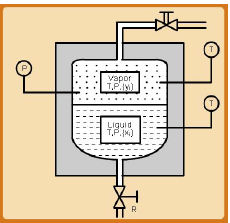Courses

# Introduction to Vapour Liquid Equilibria Civil Engineering (CE) Notes | EduRev

## Civil Engineering (CE) : Introduction to Vapour Liquid Equilibria Civil Engineering (CE) Notes | EduRev

The document Introduction to Vapour Liquid Equilibria Civil Engineering (CE) Notes | EduRev is a part of Civil Engineering (CE) category.
All you need of Civil Engineering (CE) at this link: Civil Engineering (CE)

Introduction

Both the general criterion of thermodynamic equilibrium as well as the specific condition of equality of chemical potential of each species which hold at equilibrium was introduced in the last chapter. We now develop the detailed relationships connecting the phase variables (T, P and composition) that originate from the concepts of chemical potential and the fugacity coefficient. The basic principle employed in all separation processes is that under equilibrium the compositions of phases differ from each other, and therefore it is possible to preferentially concentrate one species over another (or others) in one particular phase. This feature of phase equilibria is exploited in a wide variety of process equipments such as distillation, extraction, crystallization, etc.

This chapter focuses on the vapour-liquid equilibria (VLE) problem which is depicted schematically in fig. 7.1. When a multi-component, vapour and liquid phase – each (say) containing N chemical species – co-exist in thermodynamic equilibrium at a temperature T and pressure P, the phase compositions {y1,y2,yN-1
and { x1,x2, ...xN−1} remain invariant with time, and are related by a unique set of relations. If the relations are known as a function of temperature, pressure and compositions {yi and xi } for each species, then provided some of these variables are specified the rest may be calculated. We will derive such relations for real multi-component systems; but as in all cases of thermodynamic modeling we take the ideal system as our starting point. Next the VLE of systems at moderate pressures are treated. Finally the relations for VLE at high pressures are presented. In the following section we derive the relations that hold for pure component VLE before describing those which apply to multi-component systems.Fig. 7.1 The VLE Problem Description

Offer running on EduRev: Apply code STAYHOME200 to get INR 200 off on our premium plan EduRev Infinity!

,

,

,

,

,

,

,

,

,

,

,

,

,

,

,

,

,

,

,

,

,

;